MAA Minicourse #7 ¤
January 7 and 9, 2016
Making Sense of Calculus with Mapping Diagrams
Part I

1.Mapping Diagrams. ¤

What is a mapping diagram?
Introduction and simple examples from the past: Napiers LogarithmSee: http://www.maa.org/press/periodicals/convergence/logarithms-the-early-history-of-a-familiar-function-john-napier-introduces-logarithms

Logarithms: The Early History of a Familiar Function - John Napier Introduces Logarithms  by Kathleen M. Clark (The Florida State University) and Clemency Montelle (University of Canterbury)Linear Functions.
Understanding functions using tables. mapping diagrams and graphs.
Functions: Tables, Mapping Diagrams, and Graphs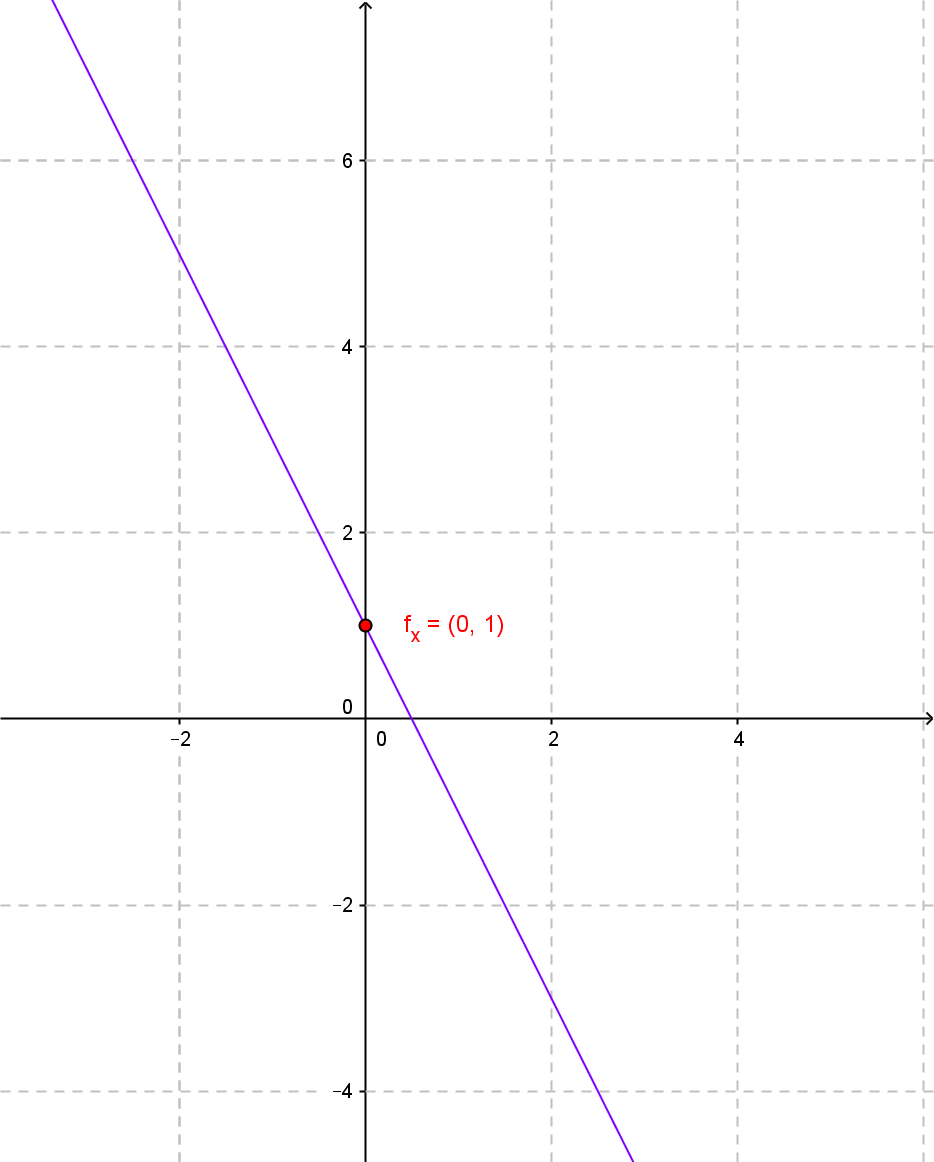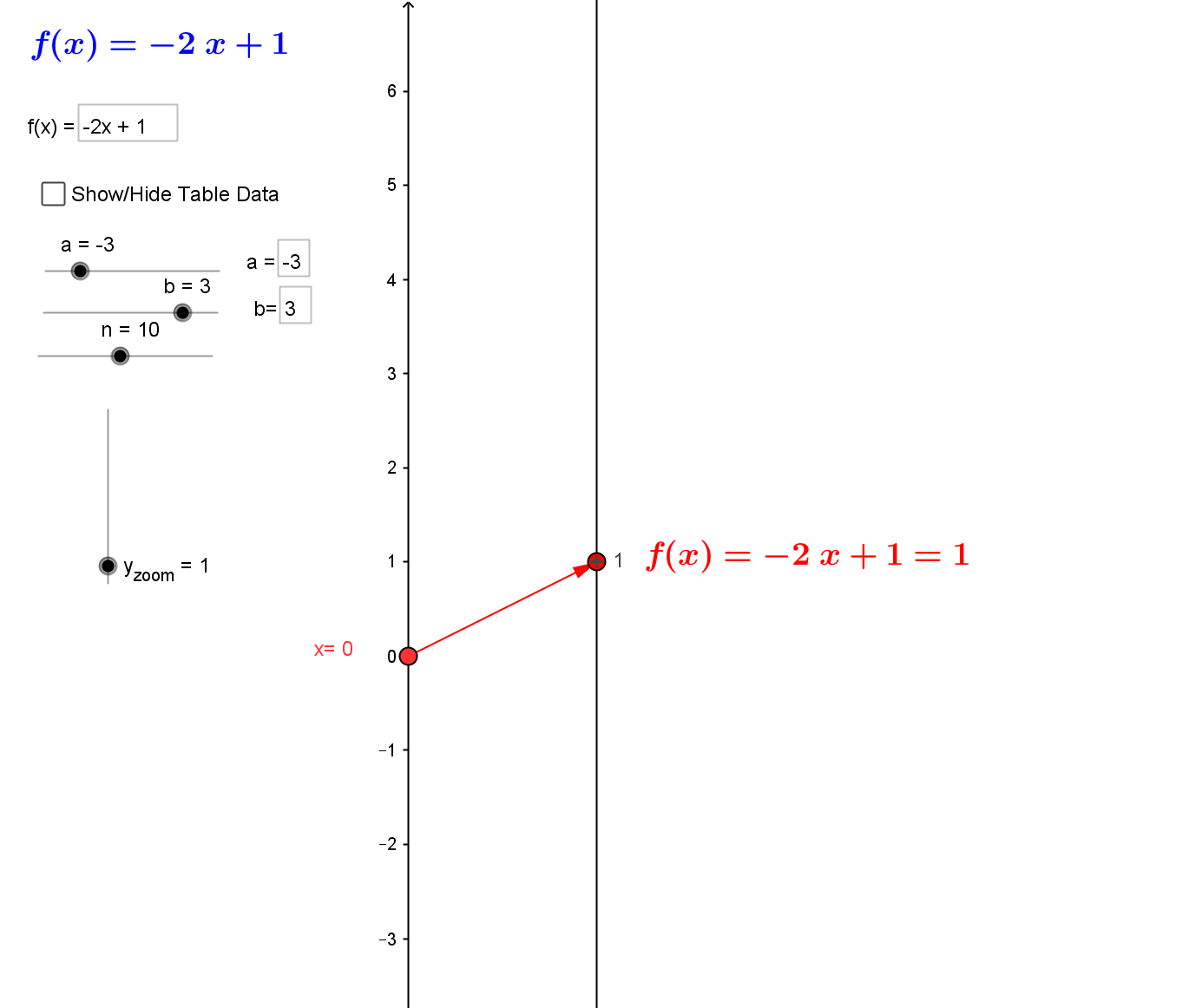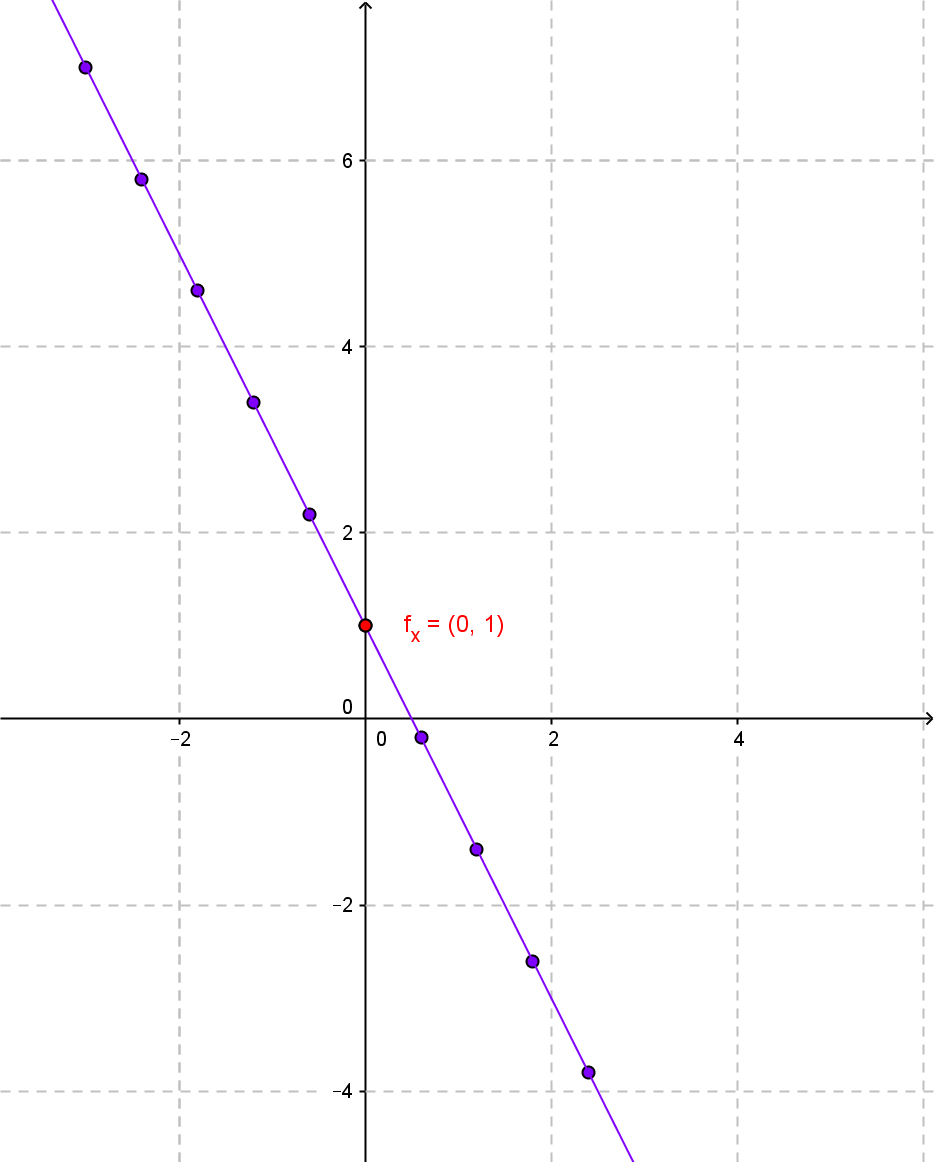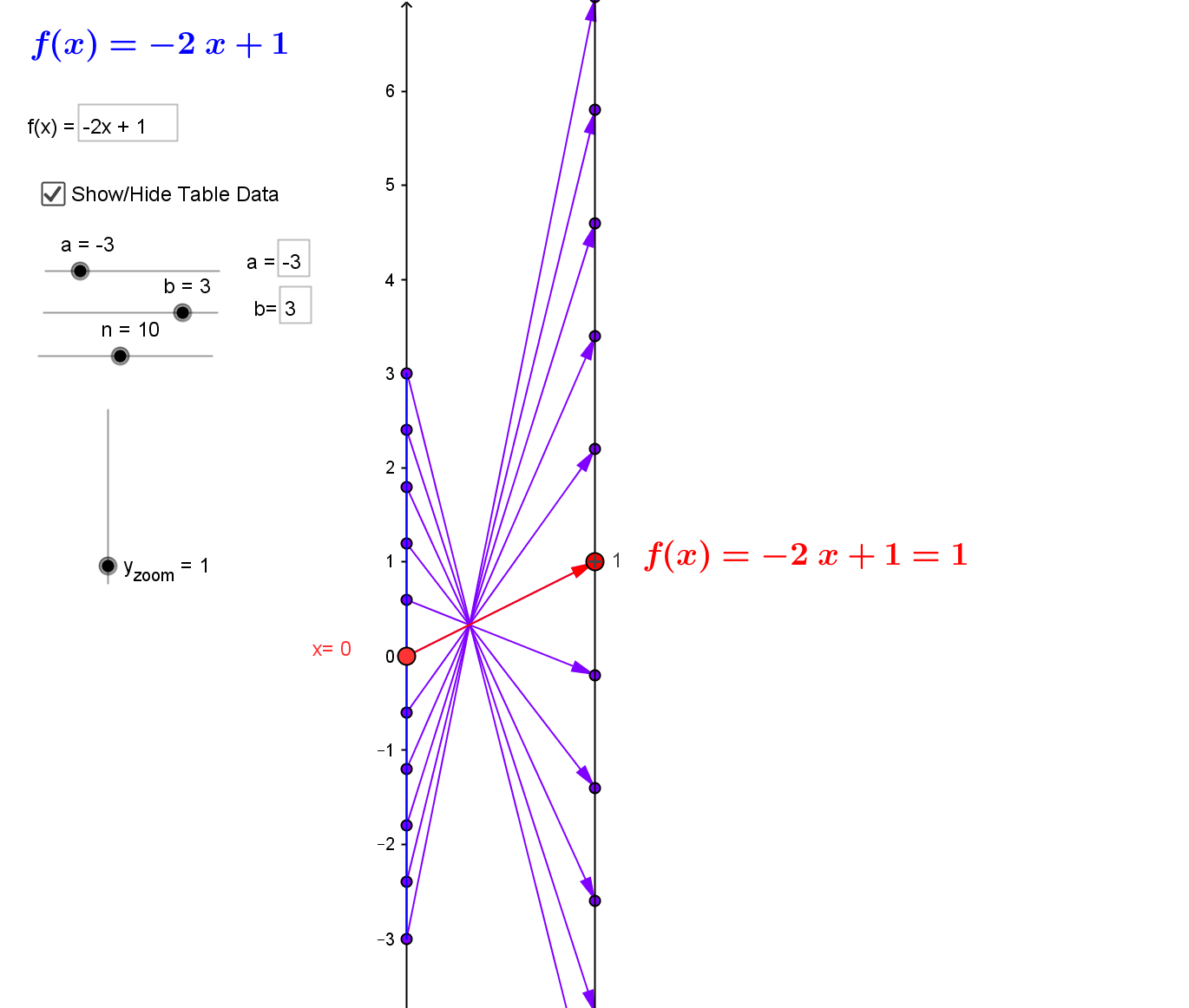2. Linear Functions. ¤
Linear functions are the key to understanding calculus.
Linear functions are traditionally expressed by an equation like :
$f(x)= mx + b$.
Mapping diagrams for linear functions have one simple unifying feature- the focus point, determined by the numbers
$m$ and $b$, denoted here by $[m,b]$
.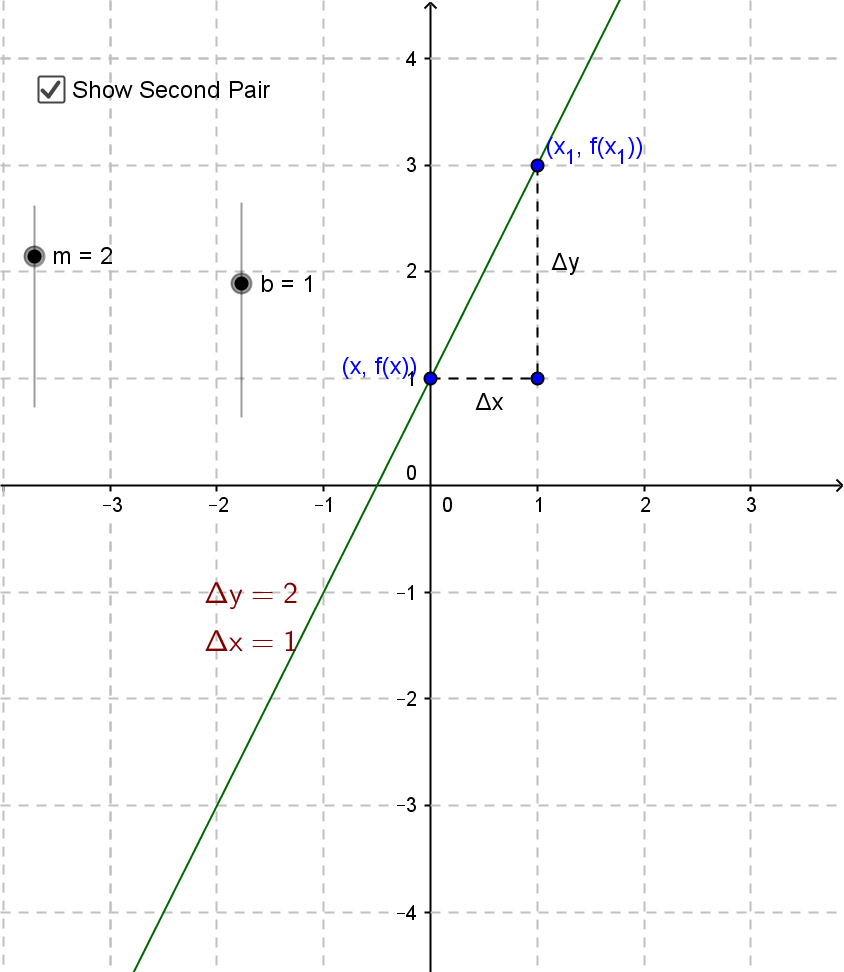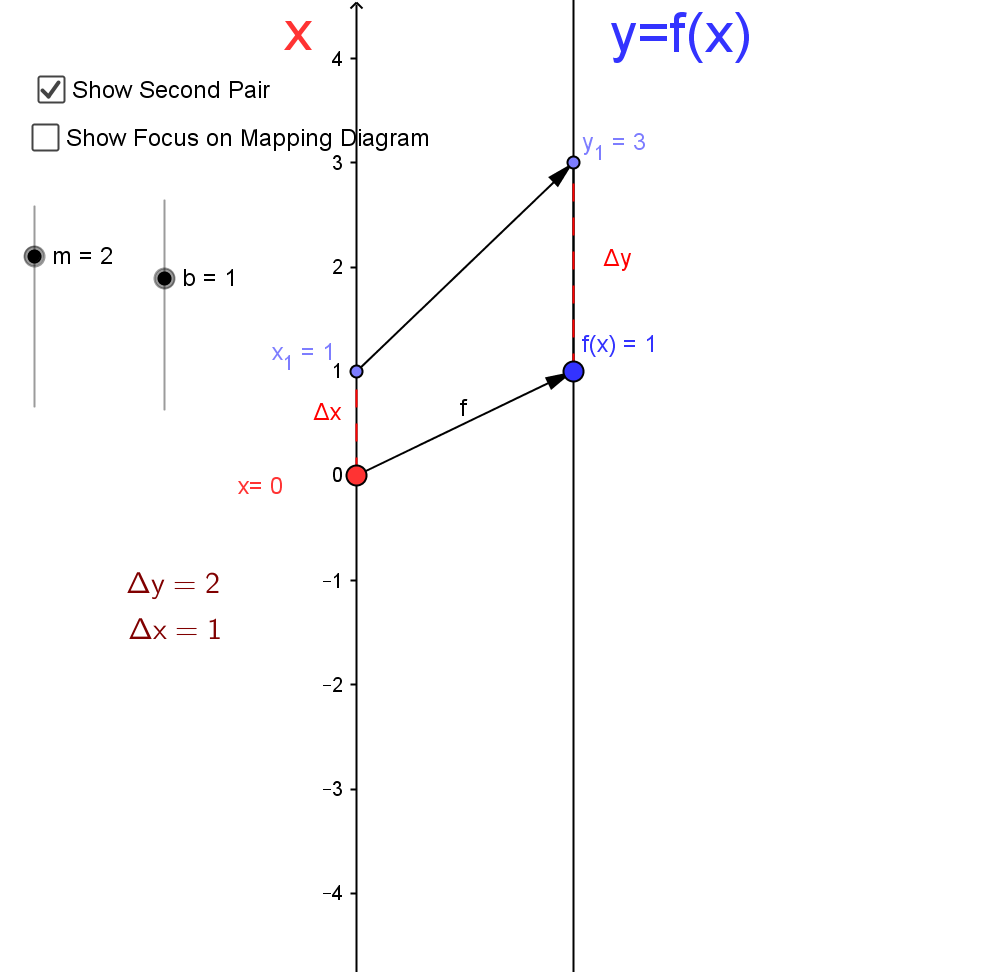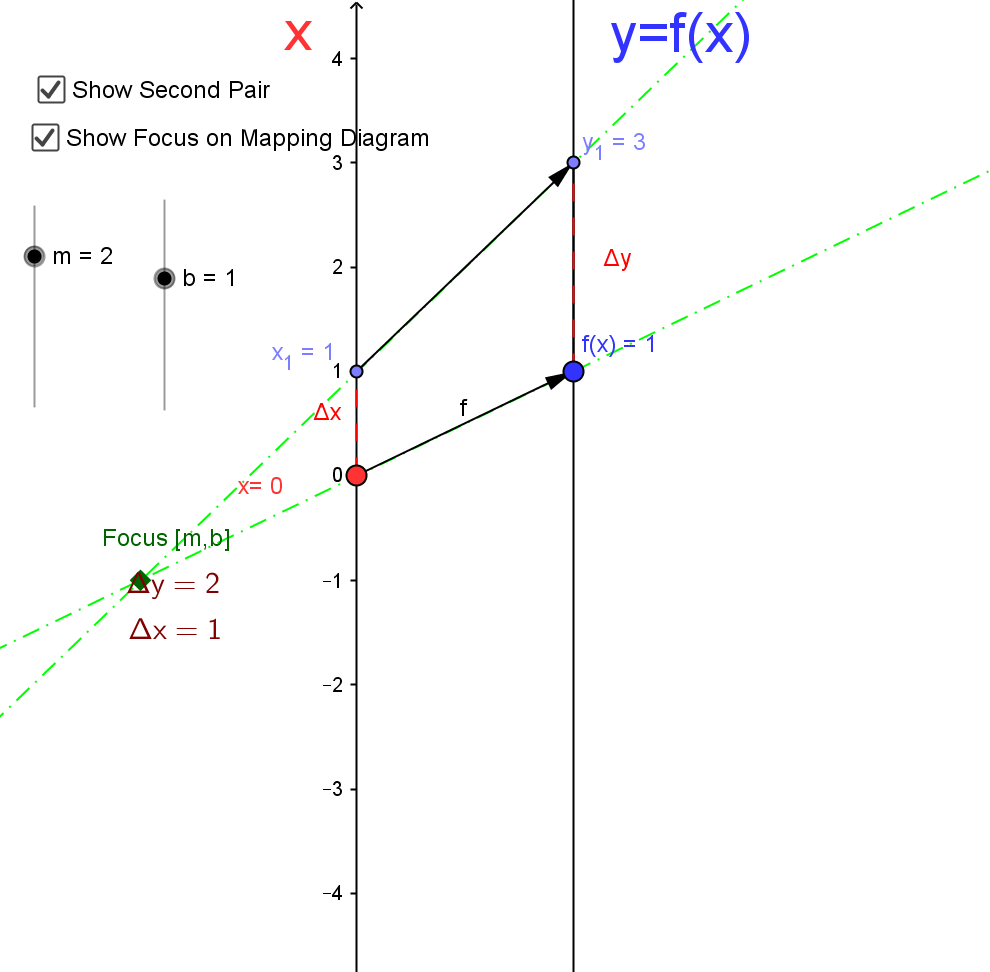Mapping Diagrams and Graphs of Linear Functions
Visualizing linear functions using mapping diagrams and graphs.
This is a Java Applet created using GeoGebra from www.geogebra.org - it looks like you don't have Java installed, please go to www.java.com
Notice how points on the graph pair with arrows and points on the mapping diagram.

3.Limits and The Derivative ¤
Mapping Diagrams Meet Limits and The Derivative
3.1 Limits with Mapping Diagrams and Graphs of Functions

The traditional issue for limits of a function $f$ is whether $$\lim_{x \rightarrow a}f(x) = L$$
.
The definition is visualized in the following example.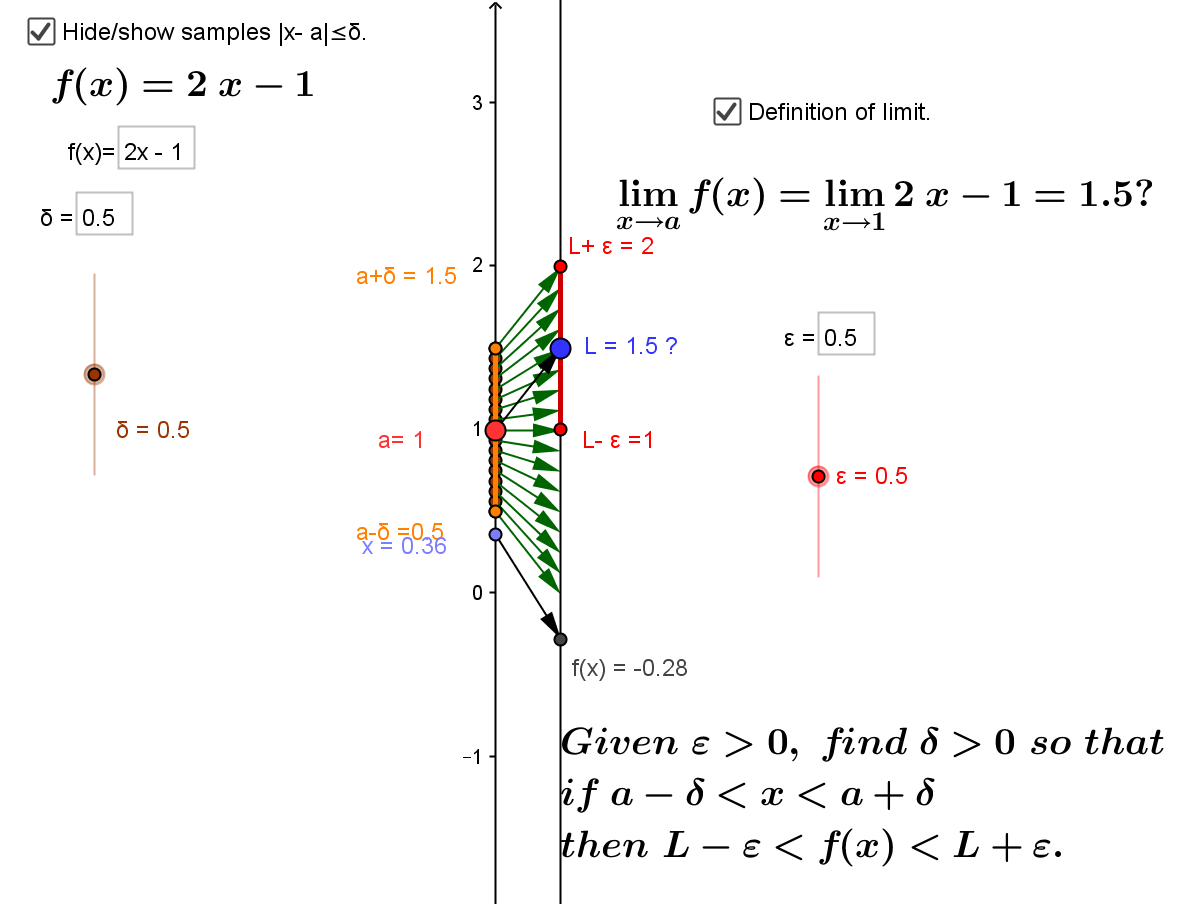Mapping diagrams and graphs visualize how the definition of a limit works for real functions.

Notice how points on the graph pair with the points and arrows on the mapping diagram.

3.2 The derivative of $f$ at $a$ is a number, denoted $f'(a)$, defined as a limit of ratios ( average rates or slopes of lines). i.e., $$f'(a) = \lim_{x \rightarrow a} \frac {f(x)-f(a)}{x-a}.$$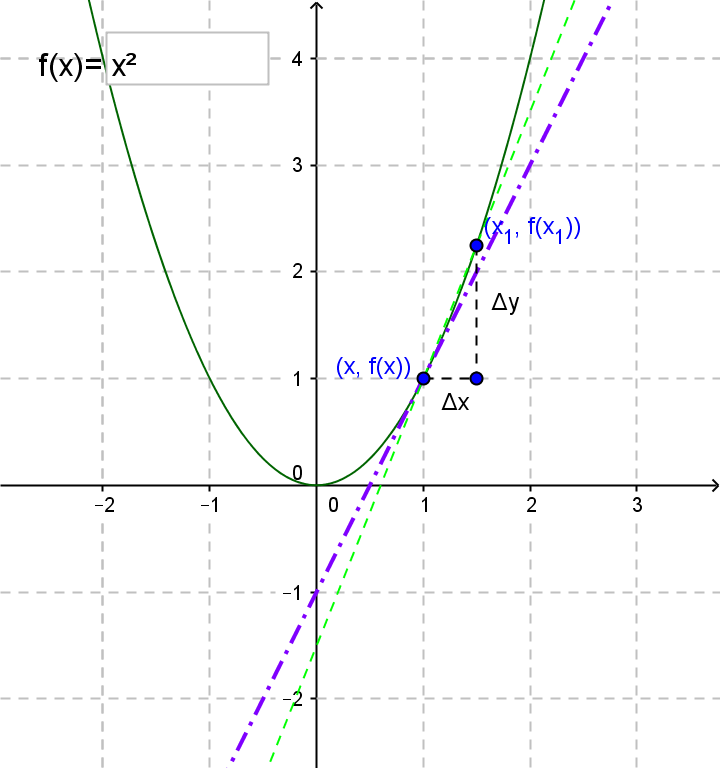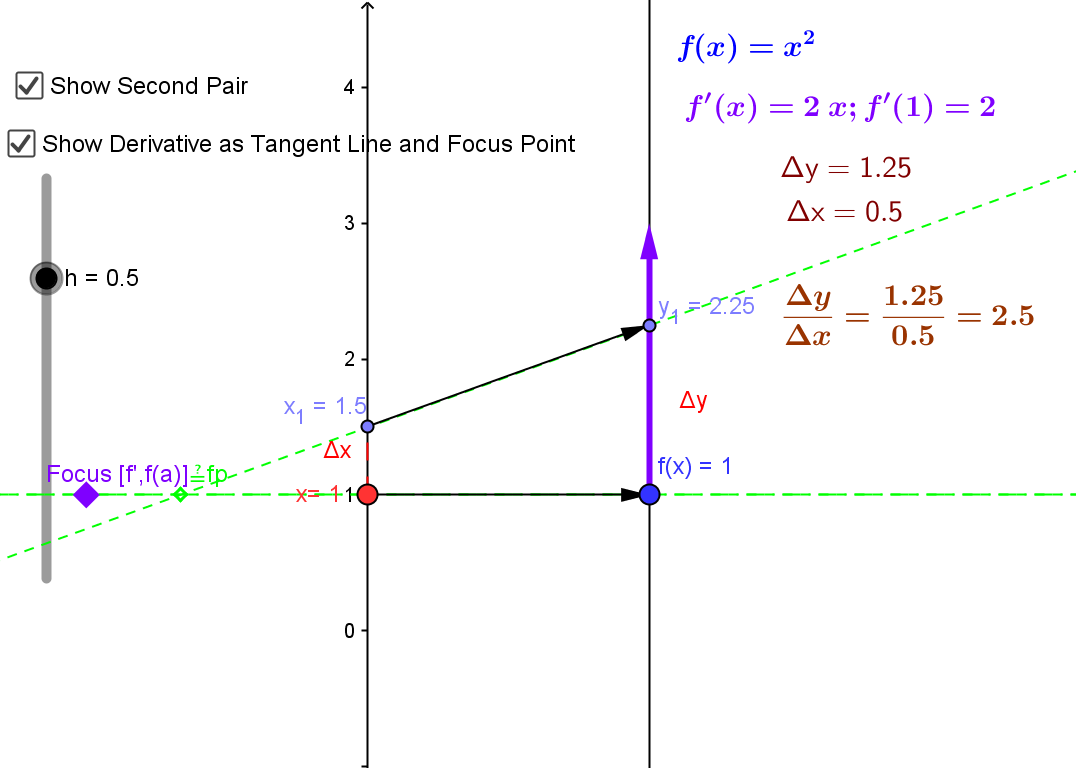The derivative can be visualized using a tangent line on a graph or a focus point and derivative "vector" on a mapping diagram.

The derivative can also be understood as the magnification factor of the best linear approximating function.¤

The derivative can be visualized using focus points and derivative "vectors" on a mapping diagram.

3.3 Mapping Diagrams for Composite (Linear) Functions ¤
This is the fundamental concept for the chain rule.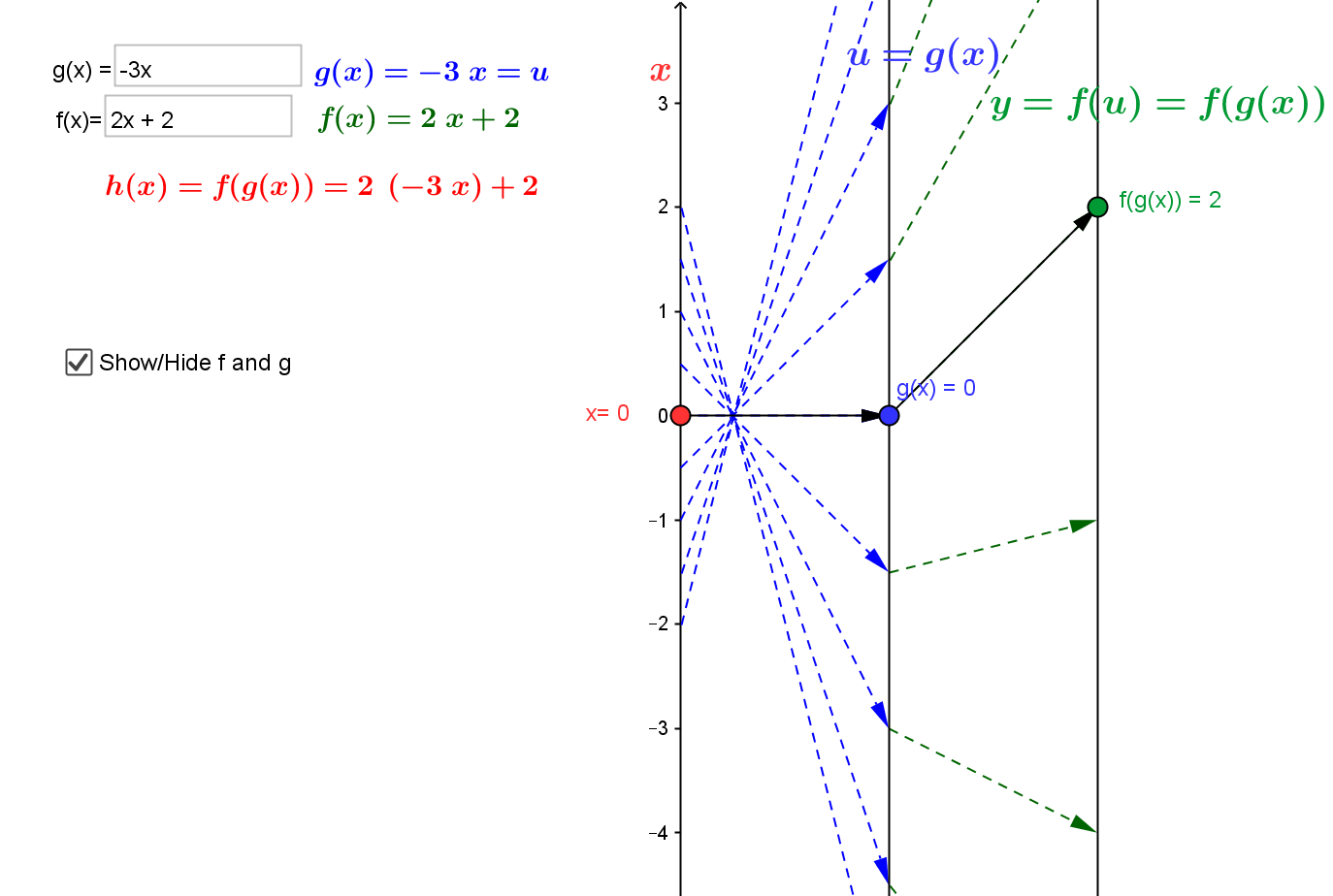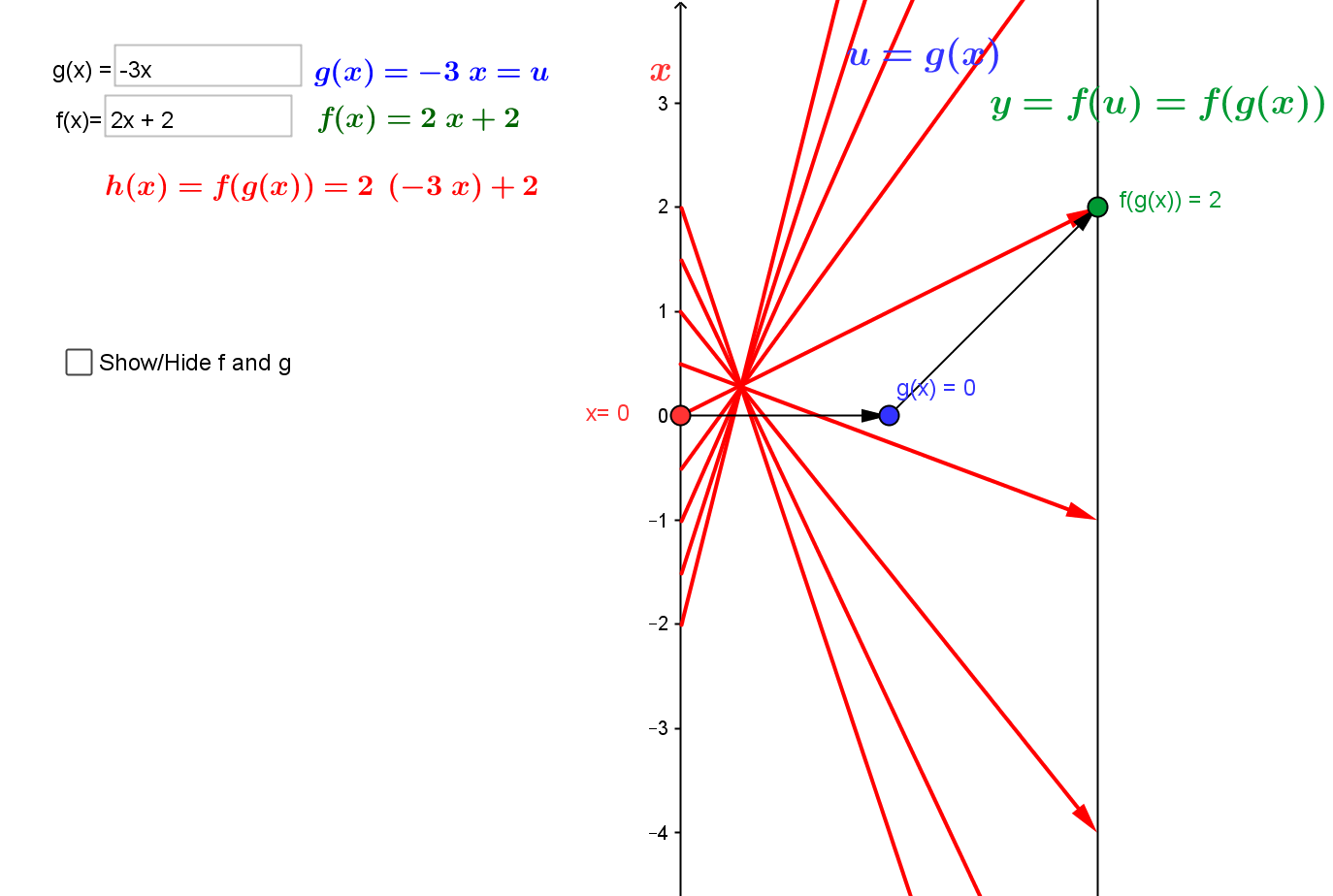Visualizing the composition of linear functions using mapping diagrams.

3.4. Intermediate Value theorem and Newton's method.

3.4.1. Continuity can be understood by connecting it to the Intermediate Value Theorem (IVT) and solving equations of the form $f(x) = 0$.
IVT: If $f$ is a continuous function on the interval $[a,b]$ and $f(a) \cdot f(b) \gt 0$ then there is a number $c \in (a,b)$ where $f(c) = 0$.
Mapping diagrams provide an alternative visualization for the IVT. They can also be used to visualize a proof of the result using the "bisection method."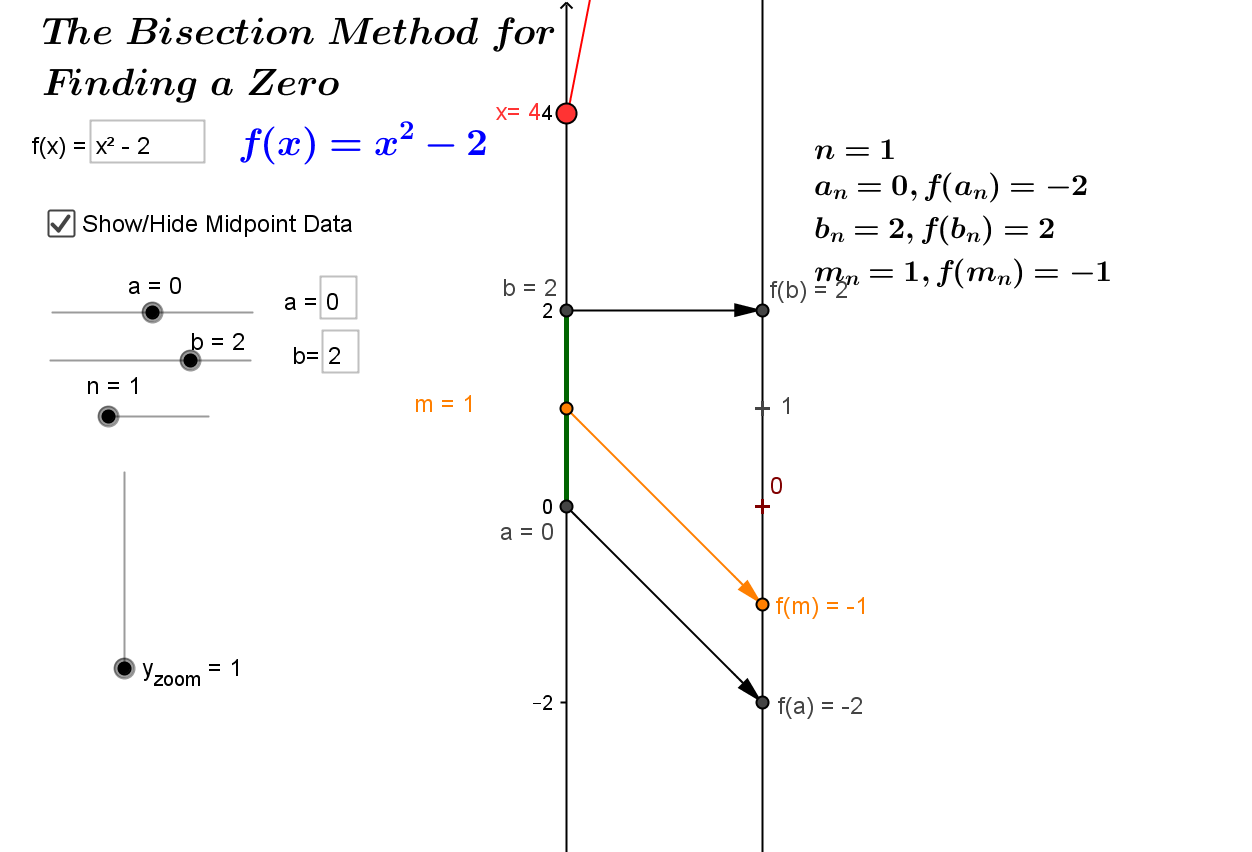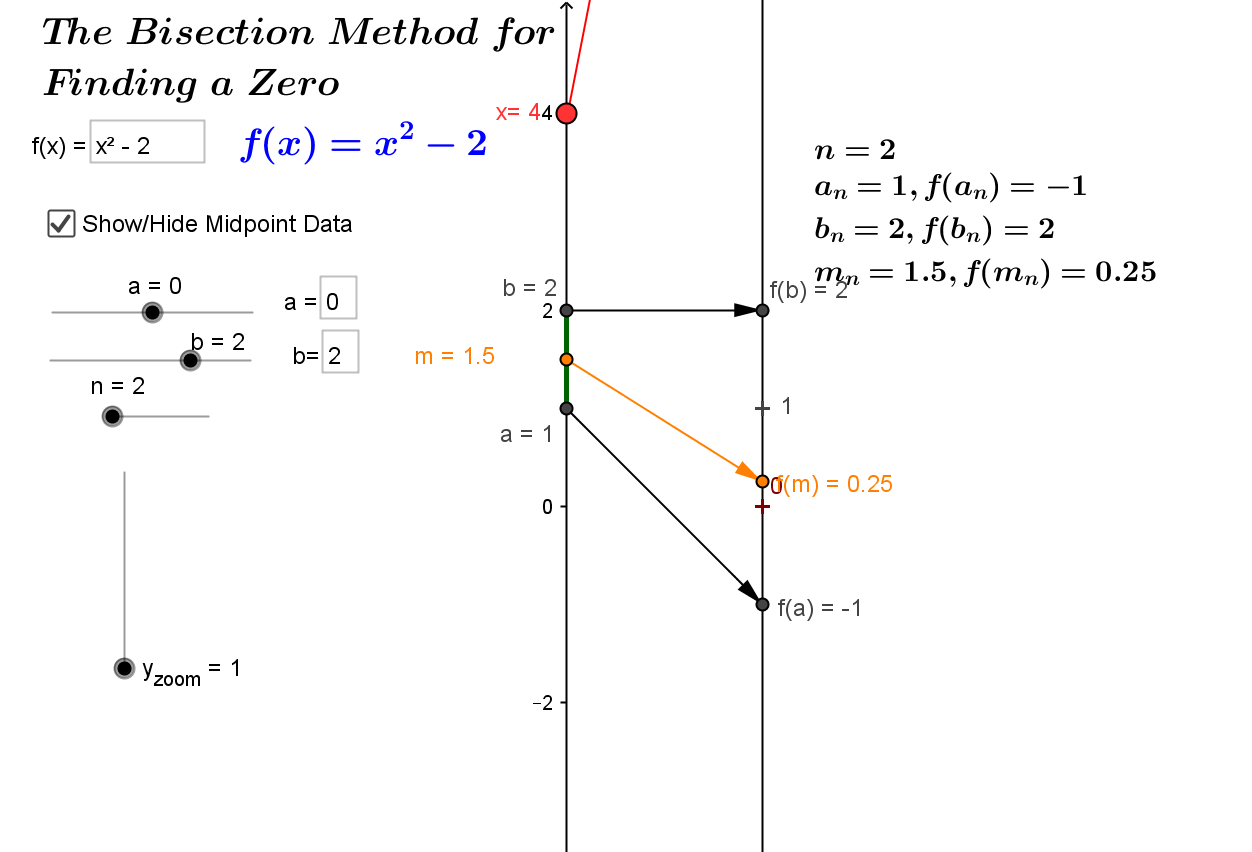Insert GEOGEBRA for Bisection and IVT.
This is a Java Applet created using GeoGebra from www.geogebra.org - it looks like you don't have Java installed, please go to www.java.com

3.4.2 An early application of the first derivative, Newton's method for estimating roots of functions is visualized with mapping diagrams.